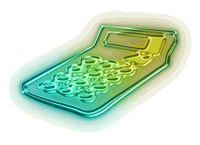# How to Calculate EPF Dividend?The calculation for EPF dividend is not straight forward. It is not calculated by simply multiply the total contribution or year end balance with the declared dividend rate.

The way EPF dividend calculated is based on daily rest. Means that, dividend is calculated based on daily balanced of each account 1 and 2. However, please bear in mind the following,

• Monthly contribution start earnings dividend only on the last day of the month irrespective the actual day the monthly contribution is deposited.
• For withdrawal, dividend for the withdrawal sum is only eligible up to the day prior to withdrawal day. No dividend will be given on the withdrawal day.

The following is simple example on how to calculate. Alternatively, you may want to use online “EPF Dividend Calculator

The formula to calculate EPF dividend is as follow,

Daily Dividend = Daily Balance x Yearly Dividend / No of Days in a Year

Total Yearly Dividend = Sum of Daily Dividends.

Take example on the following scenario,

EPF declared 5.65% dividend for year 2009. Opening balance, monthly contribution and withdrawal for Mr M in 2009 are as follow,

Items Account 1 Account 2
Opening Balance (RM) 70,000 30,000
Monthly Contribution (Jan-Dec) (RM) 700 300
Withdrawal ( 15th Feb) (RM) 1000 0

This is how to calculate the dividend received by Mr M,

#### January 2009

From 1st until 30th January, dividend is calculated as follow,

Account 1 dividend = 70,000 x 5.65% / 365 x 30 days = 325.07
Account 2 dividend = 30,000 x 5.65% / 365 x 30 days = 139.32

On 31st on January, dividend is calculated as follow,

Account 1 dividend = (70,000 + 700) x 5.65% / 365 = 10.94
Account 2 dividend = (30,000 + 300) x 5.65% / 365 = 4.69

#### February 2009

From 1st until 14th February, dividend is calculated as follow,

Account 1 dividend = (70,000 + 700) x 5.65% / 365 x 14 days = 153.22
Account 2 dividend = (30,000 + 300) x 5.65% / 365 x 14 days = 65.66

From 15th until 27th February, dividend is calculated as follow,

Account 1 dividend = (70,000 + 700 – 1000) x 5.65% / 365 x 13 days = 140.26
Account 2 dividend = (30,000 + 300) x 5.65% / 365 x 13 days = 60.97

On 28th on February, dividend is calculated as follow,

Account 1 dividend = (70,000 + 700 – 1000 + 700) x 5.65% / 365 = 141.67
Account 2 dividend = (30,000 + 300 + 300) x 5.65% / 365 = 61.58

#### March to December 2009

You have to continue doing this until December 2009. In the end you have sump up all the figures to obtain dividend for both Account 1 and 2.

Too complicated? Don’t worry. You can use online “EPF Dividend Calculator”.

Thanks to 1-million-dollar-blog readers, “marx” who shows us on how to calculate EPF dividend correctly. Read the comments on following post for reference.

•I also commented David Lee’s post: http://imdavidlee.com/how-to-calculate-your-epf-dividend/

See how I “debunk” his statement :p below
http://farm5.static.flickr.com/4077/4934130168_6892ae4afd_b.jpg

http://farm5.static.flickr.com/4122/4933536469_5559632de5_b.jpg

You take the effort to combine both the “annual” & “monthly” dividends, that’s good ! You even have the online EPF Dividend calculator now. That’s one of its kind and perhaps the ONLY ONE in Malaysia ! That’s great !

•marx,

Credit also goes to you for finding the right formula to calculate. 🙂

•I just check my 2010 EPF statement online. It is 5.8% for year 2010 dividend rate !

•You leave your MONEY in EPF RM 100K and you only get RM 464.39 per month !!!!.
What a STUPID LOL are WEEEEEEEE??????? To ALL my friends “Get out that MONEY” thru EPF investment scheme and invest in EPF UNIT TRUST either Conventional or Islamic.
Good price sell and returned back to EPF.
Invest again every 3 months and continue until your MONEY GROW like ‘DURIAN THAILAND’
Nowadays ALL MANAGERS keep on finding a way to built a BRANDNAME for themselves,where in actual “BRAIN” they are “CROOKs” and “THIEVES”. OPEN your EYES WIDE and look at “THEM” closely.Dont be temted by that BEAUTIFUL SPEECH or overwhelming presentation ada udang disebalik batu.

•I am confuse on a scenario, where if I would to return the money from investment into my epf account will I entitle for the dividend on the returning amount?

•Hi, wonder if you know about epf balance which has exceed 1 million ringgit. I heard anything above that wont be getting the full dividend but only 2.5%.

••